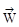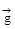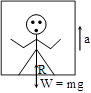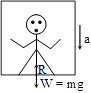# Newton's Laws Of Motion & Friction

#### 5. Motion in a lift

5. Motion in a lift
Weight :
The pull of earth on any body under its gravitational influence is called the weight of the body. This force is directed towards the centre of the earth. This force produces an acceleration on the body called the acceleration due to gravity.
If W is the weight of body of mass m, then= mNewton
Note :
The weight of mass m of a body is also taken as m kilogram weight or m kilogram force m kg weight = mg Newton
If a body is on an accelerated platform, the weight of the body appears changed and this new weight is called apparent weight. Let a man of weight
W = mg be standing in a lift.
We consider the following cases.
Case (A) :
If the lift is unaccelerated (= 0 or constant) :
In this case there is reaction R = mgHence apparent weight = actual weight
W' = Actual weight = mg
Case (B):
If the lift is accelerated upward (when= constant) :
The net forces acting on the man are⇒ R – mg = ma
∴ Apparent weight
W' = R = mg + ma
Note : Apparent weight (W') > Actual weight (mg)
Case (C) :
If the lift is accelerated downward :
Here the weight 'mg' acts downwards, while the reaction,R acts upward
Apparent weight W' = R
⇒ mg – R = ma
⇒ R = mg – ma
Note :
(i) Apparent weight W' < Actual weight W
(ii) If g = a ⇒ W' = 0
Thus in a freely falling lift, the man will experience a state of weightlessness
Case (D) :
If the lift is accelerated downwards such that a > g :
In this case ma is greater than the weight (mg)
∴ Apparent weight W' = m(g – a) = negative
There is no meaning of negative apparent weight, therefore will be zero.
So the man will be accelerated upward and will stay at the ceiling of the lift.

If you want to give information about online courses to other students, then share it with more and more on Facebook, Twitter, Google Plus. The more the shares will be, the more students will benefit. The share buttons are given below for your convenience.

#### IIT (Class X)

• Unit, Dimension & Error
• Vectors
• Motion in One Dimension
• PROJECTILE MOTION
• NEWTON'S LAWS OF MOTION & FRICTION
• WORK, POWER, ENERGY & CONSERVATION LAWS
• CIRCULAR MOTION & ROTATIONAL DYNAMICS
• GRAVITATION